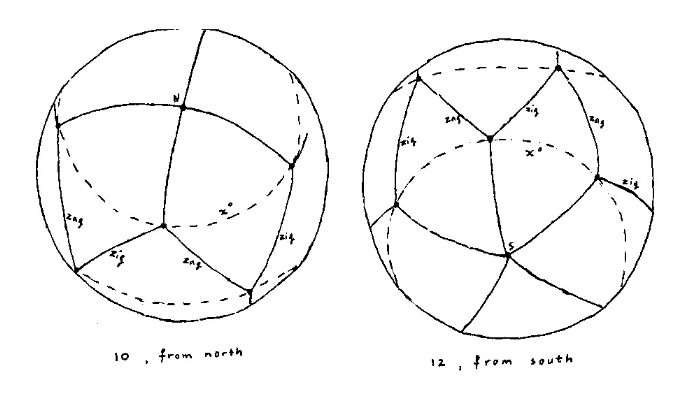Solutions Christmas Problem 2003

Find a configuration of ten (twelve) points on a sphere such that the minimal distance between two of these points is as large as possible.

Find now here the solutions of the prize winners.

MY FIRST APPROACH:

We consider a sphere with radius 1. So the answers beneath are expressed in units of one thousand kilometer.
A 'great circle' is a circle that lies upon the sphere and in a plane which contains the center of the sphere. These great circles are also called 'geodetic lines'.

The configuration with ten pirates that follows, is not yet the best one.
Within two weeks, when I write about the contributions of the competitors, I will give two configurations with ten pirates with a minimum distance that is a little bit larger.

1) In a first trial with ten pirates, I restrict myself to configurations that have a north pole and a south pole. So I begin with placing two pirates on both poles as far away from each other as possible. The other eight points then lay between x degrees northern and southern latitude, for some x that we have to determine.
The shortest paths over the sphere are the great circles of the sphere. However, since greater circle arcs bring greater chords and vice versa, the optimal configuration is the same if one measures distances through space and if one measures them along great circles of the sphere.
We can divide the region between x degrees northern and southern latitude in eight congruent "quadrilaterals". Every such quadrilateral is the region on the sphere confined between the circles at x degrees northern and southern latitude and the meridians at (k-1)*45 and k*45 degrees eastern longitude (k=1,2,..,8).
For an optimal configuration, the eight points have to be chosen alternatingly left beneath and right above in the quadrilateral, such that the lines of connection are (geodetic) diagonals of the quadrilaterals, forming together a zigzag pattern. Otherwise, there are always two points closer in distance than necessary. So four points form (in space) a square at x degrees northern latitude, and four another one at x degrees southern latitude, and the second square is rotated over 45 degrees with respect to the first.

The ten points then have the following coordinates: (with t:=(90-x)*pi/180)
(0,0,1),
(sin(t),0,cos(t)), (0,sin(t),cos(t)), (-sin(t),0,cos(t)), (0,-sin(t),cos(t)),
(sqrt(2)*sin(t)/2,sqrt(2)*sin(t)/2,-cos(t)), (-sqrt(2)*sin(t)/2,sqrt(2)*sin(t)/2,-cos(t)),
(-sqrt(2)*sin(t)/2,-sqrt(2)*sin(t)/2,-cos(t)), (sqrt(2)*sin(t)/2,-sqrt(2)*sin(t)/2,-cos(t)),
(0,0,-1).

We measure the distance between any two points following the great circle through those points. The distance is equal to the angle (in radials) between the vectors from the center (0,0,0) of the sphere to the points. We calculate this angle using the inner product.
There are three distinct distances between neighbour points:
1) t (between a pole and another point in the same hemisphere)
2) arccos(cos2(t)) (between two points in the same square)
3) arccos(sqrt(2)*sin2(t)/2 - cos2(t)) (between neighbour points from distinct squares).

Graphical analysis learns that the minimum of distances 1), 2) and 3) is maximal for the value of t for which 1) equals 3).
Then we find:
cos(t) = sqrt(2)*sin2(t)/2 - cos2(t),
so cos(t)(1+cos(t)) = sqrt(2)*(1-cos2(t))/2,
so cos(t) = sqrt(2)*(1-cos(t))/2,
so cos(t) = sqrt(2)/(2+sqrt(2)) = 1/(1+sqrt(2)), so the minimum distance then is t = arccos(1/(1+sqrt(2))) = 1.143717740 .2) There is a platonic solid with twelve points. This platonic solid has thirty edges and twenty faces. All faces are congruent equilateral triangles, corresponding to congruent geodetic triangles on the sphere. These triangles cover the sphere, so this is the optimal configuration. If we choose a different configuration, there are always two points closer in distance than the length of the sides of such a triangle.
This solid has a north pole and a south pole. We then find the configuration in a way similar to that with ten pirates (see the picture). One difference is that now five points are sitting on the same circle of latitude, instead of four. Another difference is that, with twelve pirates, the smallest distance between two points on the same latitude is equal to the distance from such a point to the nearest pole, but with ten it is larger than the distance to the nearest pole.

The distance over the sphere between neighbour points is equal to the angle t for which
the distance through space between (0,0,1) and (sin(t),0,cos(t))
equals
the distance through space between (sin(t),0, cos(t)) and (sin(t)*cos(2*pi/5),sin(t)*sin(2*pi/5),cos(t)).

This angle can be calculated as follows, using Pythagoras:
sqrt(sin2(t)+(1-cos(t))2) = sin(t)*sqrt(sin2(2*pi/5)+(1-cos(2*pi/5))2),
so sqrt(2-2*cos(t)) = sin(t)*sqrt(2-2*cos(2*pi/5)),
so 2*sin(t/2) = 2*sin(t/2)*cos(t/2)*2*sin(pi/5),
so cos(t/2) = 1/(2*sin(pi/5)).
So the minimum distance is now t = 2*arccos(1/(2*sin(pi/5))) = 1.107148718 .

After the act, searching on internet, I first found this site. It seemed to indicate that both configurations that I gave above are optimal, so the one with ten pirates too.
Lateron however, searching more information, I found different configurations related to publications of Tóth (1943) and Danzer (1963). The site I found is this one.
It turns out that my first approach, starting with a north pole and a south pole, does not for many distinct numbers of pirates lead to the very best configuration. K.Schütte found in 1950 for ten pirates a complicated configuration with a minimum distance that is slightly greater (the difference is less than one percent). Then in 1963, Ludwig Danzer proved that this configuration is optimal. But only he and a few 'specialists' in the field understand how this is possible.
Professor Danzer is one of the contestants in this Christmas prize competition. He sent a reprint of the beautiful Habilitationsschrift he wrote forty years ago.
For twelve pirates, my configuration is the same as Danzer's. Notice that a platonic solid does not always give the optimal configuration. For instance, with twenty pirates it doesn't.

THE SOLUTIONS OF THE PRIZE WINNERS WILL BE PUBLISHED ON THIS SITE WITHIN TWO WEEKS.